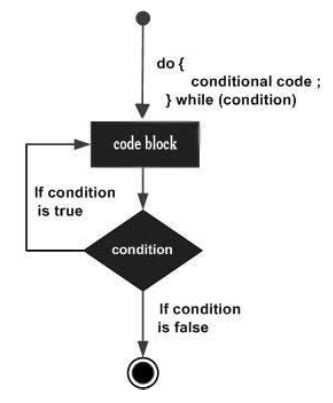# TypeScript - doŌĆ”while loop

The doŌĆ”while loop is similar to the while loop except that the do...while loop doesnŌĆÖt evaluate the condition for the first time the loop executes. However, the condition is evaluated for the subsequent iterations. In other words, the code block will be executed at least once in a doŌĆ”while loop.

## Syntax

```do {
//statements
} while(condition)
```

## Flowchart## Example: doŌĆ”while

```var n:number = 10;
do {
console.log(n);
n--;
} while(n>=0);
```

On compiling, it will generate following JavaScript code −

```//Generated by typescript 1.8.10
var n = 10;
do {
console.log(n);
n--;
} while (n >= 0);
```

The example prints numbers from 0 to 10 in the reverse order.

```10
9
8
7
6
5
4
3
2
1
0
```
typescript_loops.htm